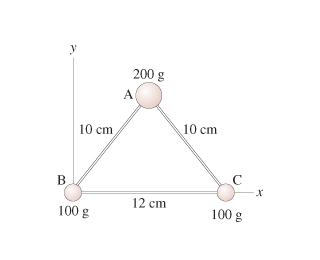# Problem: Find the moment of inertia about an axis that passes through masses B and C.Express your answer to two significant figures and include the appropriate units.

###### FREE Expert Solution

Moment of inertia of a point mass, located a distance, r, from the axis of rotation is

$\overline{){\mathbf{I}}{\mathbf{=}}{\mathbf{m}}{{\mathbf{r}}}^{{\mathbf{2}}}}$

The location of mass A from the axis of rotation is determined using the Pythagoras theorem. Distance, r, is equal to the height of the triangle.

88% (186 ratings)###### Problem DetailsFind the moment of inertia about an axis that passes through masses B and C.

Express your answer to two significant figures and include the appropriate units.